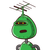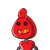# A drum contain 6 kg of rice. From this 1500 gm taken out for cooking. Find the ratio of the amount taken out to the amount left in

A drum contain 6 kg of rice. From this 1500 gm taken out for cooking. Find the ratio of the amount taken out to the amount left in the drum.​

### 2 thoughts on “A drum contain 6 kg of rice. From this 1500 gm taken out for cooking. Find the ratio of the amount taken out to the amount left in”

1.## 1:3

Step-by-step explanation:

Left in the drum=

6kg = g

1 kg = 1000 g

6kg = 6 × 1000

=6000g.

$$= 6000 – 1500 \\ = 4500g$$

### =Ratio is

1500 : 4500 [simplest form]

$$\frac{1500}{4500} \: = \: \frac{1}{3}$$

### So,ratio is 1:3.

2.